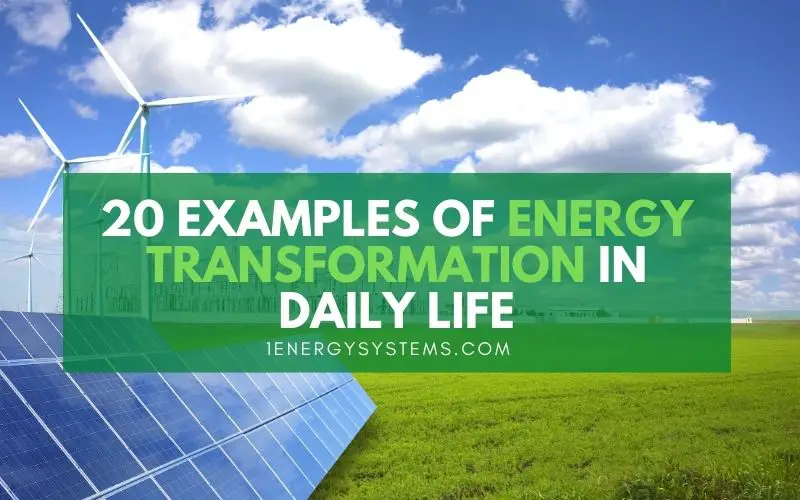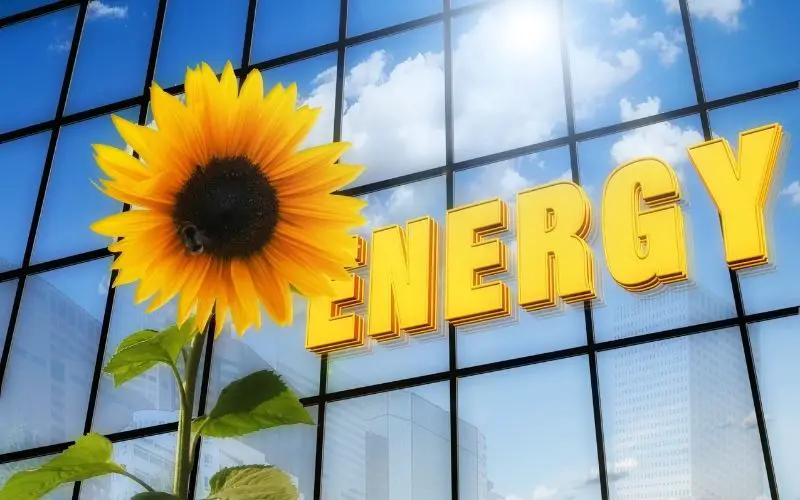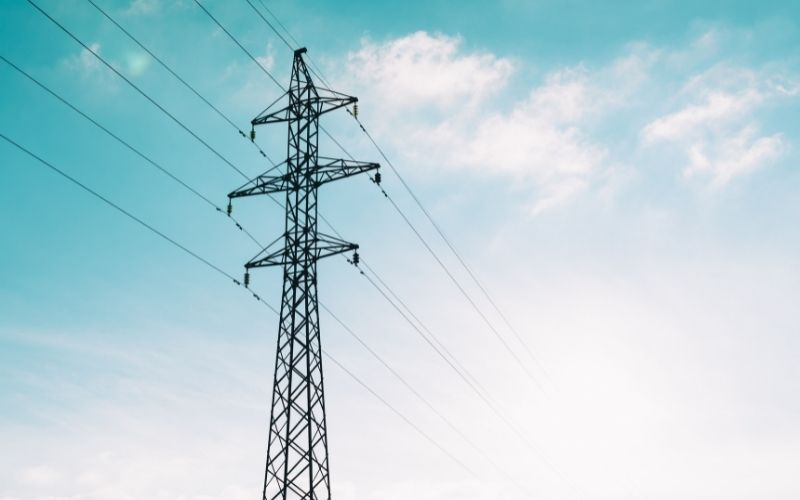# 20 Examples Of Energy Transformation In Daily LifeWe are always in pursuit of more energy to meet our growing power demands. Do you know that it is impossible to create energy?

The law of conservation of energy tells us that energy can neither be created nor destroyed. Energy can only be converted from one form to another. This law applies to any closed system, including the universe.

This means the total energy present in the universe is constant. This begs the question, “What about the numerous methods we use for energy generation? Such as in thermal power stations, nuclear power plants, or solar energy systems.”

You would be surprised to know that all these methods we use to produce electricity follow the law of conservation. Even when we say we “generate” energy, we are actually converting energy from one form to another. In most instances mentioned above, multiple conversions happen so that we end up with electrical energy.

It is not only in massive power stations that energy transformations happen. Even lifting something from the ground or consuming food involves energy transformation. It is happening with and without our knowledge, every moment of our lives.

Are you curious to know more about energy transformation? Read on. This article will explain the kinds of common energy transformations occurring in our everyday life. You will also find here examples of such conversions.

## Why is energy transformation necessary?

According to the law of conservation of energy, the total amount of energy in the universe is constant. Energy cannot be created or destroyed. However, it is possible to convert energy from one form to another.

Consider this situation. We need electrical energy to power our homes, run factories, hospitals, and offices, for transportation, communication, and entertainment. This list goes on. Where will the electricity come from?

Depends on the fuel used in the generation of electricity. In thermal power stations, coal is burned to produce electricity. In nuclear power plants, energy is generated from nuclear fission. When using solar panels, sunlight is the source of energy, while wind powers wind turbines to generate electricity.

In each one of the above instances, one form of existing energy is converted into electrical energy, often undergoing multiple transformations. For example, in thermal power stations, the chemical energy in coal is burned and transformed into heat energy. This heat energy is used to boil water and produce steam, which turns the generator turbine to produce electricity.

Another common example is deriving energy from the food we eat. To perform our activities and run our bodily functions, we need energy. We get this energy from food. The food contains chemical energy which gets converted into thermal and mechanical energy. We need thermal energy to maintain our body temperature and mechanical energy to perform work.

Energy is present in nature in various forms – as chemical energy in various things we use as fuels, as solar energy in sunlight, as wind energy, as kinetic energy, as gravitational potential energy, and so on.

Without energy transformation, it would be impossible for us to carry out any work. If conversion of energy is not possible, what is the use of electrical energy? After all, we use electricity to get some work done. Such as operating an appliance or a device, lighting a bulb, working laptops and mobile phones, making machines work, or running cars and trains. In each one of these applications, electrical energy is converted into some useful form or the other.

The ability to convert energy from one form to another helps us meet our energy demands.## Energy transformation to generate electricity

As we use various methods to produce electricity, let’s see how energy transformation happens in each instance.

### 1. Solar energy system

Sunlight is the input energy here. It consists of both light energy and heat energy. Solar panels use light energy to generate electricity.

Related:

### 2. Wind turbine

The wind turbines use the kinetic energy in the moving wind to produce mechanical energy to turn the turbines, which in turn generates electricity.

Related:

### 3. Hydroelectric station

The potential energy in the stored water in the dam is converted to kinetic energy when the gravitational force acts on it. When the falling water is used to turn a turbine, kinetic energy is converted into mechanical energy. Finally, a generator converts mechanical energy into electricity.

Related:

### 4. Biomass

Biomass is burned in a container to convert the chemical energy contained in it into heat energy. The heat is used to produce steam, which in turn turns the turbine. The mechanical energy is converted into electricity by the generator.

### 5. Geothermal energy plant

The hot water from deep underground is pumped to the surface where it gets converted into steam. This heat energy is used to turn a turbine to produce mechanical energy. The generator used mechanical energy to generate electricity.

### 6. Thermal power station

Here, the chemical energy in coal is converted to heat energy, which is then used to produce steam by boiling water. The steam is used to turn the turbines which involve converting heat energy into mechanical energy. The generator converts mechanical energy into electrical energy.

### 7. Nuclear power plant

During nuclear fission, nuclear energy is converted into heat energy. Just like in a thermal power station, the heat energy is used to produce steam to turn turbines. The converted mechanical energy is used in the generator to generate electricity.

Related:## Everyday energy conversion examples

Electricity is merely one form of energy, which is converted into other energy forms to get things done. Most of the energy transformations happening in our daily life involve the conversion of electricity into useful energy forms.

### 8. Air conditioner

In this, electricity is used to transport heat from one place to another. This is managed through the conversion of electrical energy to kinetic energy. The refrigerant absorbs the heat energy inside the room and transports it outside. Once cool, refrigerant is again circulated back inside to carry more heat outside.

### 9. Television

A television converts electrical energy into light and sound energies.

### 10. Incandescent bulb

Converts electrical energy into light energy. However, some of the electrical energy is converted into heat energy and lost in the process.

### 11. Table/ceiling fan

Electrical energy is converted into mechanical energy. The by-products are heat and sound energy.

### 12. Electric motor

A motor is just the polar opposite of a generator. A motor converts electrical energy into mechanical energy. The by-products of this process are heat and sound energies.

Whenever there is a by-product during energy transformation, some of the energy is lost or wasted. This is an indication of the inefficiency of the process.

### 13. Vehicles

When fossil fuels like petrol or diesel are used to run vehicles, the chemical energy contained in them is converted into mechanical energy. Heat energy is also generated as a by-product.

Related:

### 14. Food we eat

Chemical energy is stored in the food, which is converted into mechanical energy by our body. The by-product of heat energy is, in fact, useful for us to keep our bodies warm.

### 15. Dry cell

When connected, a dry cell converts the chemical energy contained in it to produce electrical energy. Often heat energy is a by-product of the process.

### 16. Gas stove

A gas stove uses natural gas, syngas, butane, propane, or liquified petroleum gas (LPG) as fuel to cook food. Here the energy transformation is from chemical energy to heat energy.

### 17. Firecrackers

When a firecracker is lit, it turns the chemical energy in it to light, sound, heat, and mechanical energy.

## More energy transformations

### Mechanical energy

Unlike other forms of energy, mechanical energy occurs in two types – potential energy and kinetic energy. While potential energy is the energy of an object by virtue of its position, kinetic energy is an object’s energy by virtue of its motion. To convert mechanical energy into other forms, potential energy needs to be converted into kinetic energy.

### 18. Generator

It converts the kinetic mechanical energy of the turbine to generate electricity. The by-products are heat and sound.

### 19. Photosynthesis (Light energy)

The trees and plants use the light energy in the sunlight to produce food for us. The energy is stored in food as chemical energy.

### 20. Microphone (Sound energy)

When we speak into a microphone, the sound energy gets converted into electrical energy. This is converted back into sound energy by the speaker.

#### Bottom line

The sun is the main source of energy for us – whether we are directly using solar energy to generate electricity using PV cells or the trees use it to produce food for us to eat. Do you know where the sun gets its energy from?

Nuclear fusion is the source of the sun’s energy. Energy is generated by the nuclear fusion of hydrogen nuclei into helium. The sun converts nuclear energy into heat and light energy and it reaches us in the form of sunlight.

We rarely think about energy transformation when we go about our daily lives. When we rub hands together to warm them, we are, in fact, converting mechanical energy into heat energy. When you strike a drum, mechanical energy turns into sound energy.

When lightning strikes, electrical energy is changed into light and heat energies. When we switch on a light, electrical energy converts into light energy.

Energy is being converted from one form to another constantly in the universe. These are only a few energy transformation examples from your daily life. Look around you and you will find energy conversion of some sort in every single activity happening around you.